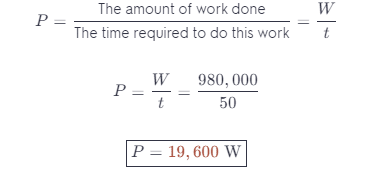# How Much Work Does An Elevator Motor Do To Lift A 1000 Kg Elevator A Height Of 100 M ?

We thoroughly check each answer to a question to provide you with the most correct answers. Found a mistake? Let us know about it through the REPORT button at the bottom of the page.

a. How much work does an elevator motor do to lift a 1000 kg elevator at a height of 100 m at a constant speed?

b. How much power must the motor supply to do this in 50 s at constant speed?

Contents

W = 980,000 J

P = 19,600 W

## Explanation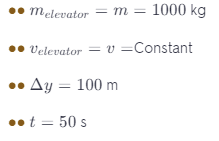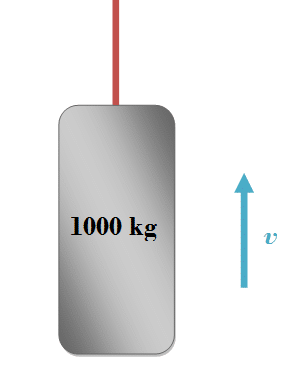We know that the work is equal to the force applied times the distance traveled.

Because the elevator is moving at a constant speed, so the net force that is acting on the elevator is zero.

This means that the Force of the motor (applied force) is equal to the weight of the elevator.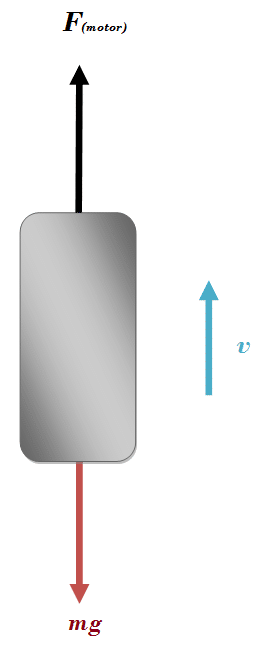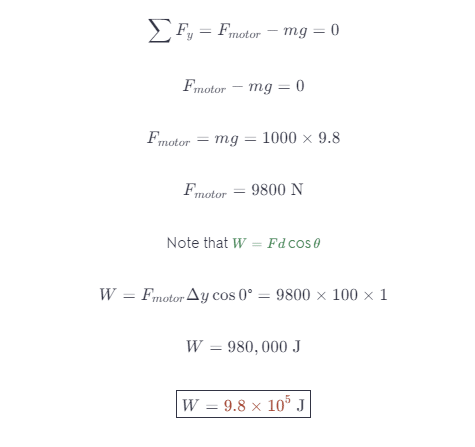We know that the power is equal to (the amount of work done) over (the time required to do this work):